Journal of Vibroengineering

Published: 30 June 2019

# Inference of the optimal probability distribution model for geotechnical parameters by using Weibull and NID distributions

Fengqiang Gong1
Tiancheng Wang2
Shanyong Wang3
1, 2School of Resources and Safety Engineering, Central South University, Changsha, Hunan, 410083, P. R. China
3ARC Centre of Excellence for Geotechnical Science and Engineering, Faculty of Engineering and Built Environment, The University of Newcastle, Callaghan, New South Wales, 2308, Australia
Corresponding Author:
Fengqiang Gong
Views 249

#### Abstract

To obtain the optimal probability distribution models of geotechnical parameters, the goodness of fit of the normal information diffusion (NID) distribution and Weibull distribution were investigated and compared for actual engineering samples and Monte Carlo (MC) simulated samples. Two datasets from actual engineering parameters (the strength of a rock mass and the average wind speed) were used to test the fitting abilities of these two distributions. The results show that the parameters of the NID distribution are easily estimated, the Kolmogorov-Smirnov (K-S) test results of the NID distribution are smaller than those of the Weibull distribution, and the NID distribution curves can perfectly reflect the stochastic volatility of geotechnical parameters with small sample sizes. The sample size effects on the fitting accuracy of the NID distribution and Weibull distribution were also investigated in this paper. Eight simulated samples with different sample sizes, namely, 15, 20, 30, 50, 100, 200, 500, and 1000, were produced by using the MC method based on two known Weibull distributions. The results show that with an increase in the sample size, the K-S test results of the NID distribution gradually decrease and tend to converge, while the chi-square test results of the NID distribution remain low and are always lower than those of the Weibull distribution. The cumulative probability values of the NID distribution are larger than those of the Weibull distribution and are always equal to 1.0000. Finally, the comparison of the fitting accuracy between the NID distribution and normalized Weibull distribution was also analyzed.

## 1. Introduction

Due to the natural attributes of rock materials, the complexity of the geological environment and the randomness of external loading (such as impact loads, seismic response, vibratory action, etc.), uncertainty is inevitable in geotechnical engineering [1-3]. To quantitatively evaluate the influence of this uncertainty, reliability analysis has been widely used in many fields of geotechnical engineering [4, 5], such as slope reliability [6-12], tunnel and underground cavity reliability [13, 14], etc. In the reliability analysis of geotechnical engineering under quasi-static loads or vibrations loads, the inference of optimal probability density function (PDF) or cumulative distribution function (CDF) of a geotechnical parameter is one of the most essential tasks; this is the first step in a reliability analysis and plays a central role in ensuring the precision and accuracy of the geotechnical reliability analysis [15, 16]. Through the comparison and selection of the classical distributions (normal distribution, log-normal distribution, Weibull distribution, gamma distribution, etc.), some previous studies have shown that many geotechnical parameters will accept a Weibull distribution as the optimal PDFs [17-20]. However, there are some unsolved issues in the application process of the Weibull distribution. The specific PDF forms of the Weibull distribution are not uniform (including the two-parameter, three-parameter and mixed Weibull distributions), and the parameters of the Weibull distribution, such as the shape parameter $m$, the scale parameter $\sigma$ or the position parameter $\mu$, are sometimes difficult to estimate. In addition, the total cumulative probability value of the Weibull distribution is generally less than 1.0000 because its defined interval does not match the actual finite interval of geotechnical parameters. It is necessary to study the inference method, which more accurately represents the actual distribution.

In recent years, the normal information diffusion (NID) theory has been the focus of the attention of many scholars and has been further developed by C. F. Huang et al. [21, 22]. NID theory provides a new way to study function approximation based on the information assignment method of a fuzzy set. In NID theory, the original information is directly transferred to the fuzzy relation in a way that avoids calculation of the membership function and preserves the original information contained in the original data as much as possible. Due to the advantages of the information diffusion principle, NID theory has been successfully applied to some fields of study, especially to natural disaster and risk assessment [23-25].

In this paper, NID theory was introduced to fit the optimal PDFs or CDFs of geotechnical parameters. Two geotechnical parameters, the strength of a rock mass affected by acid  and the average wind speed , were used as examples to investigate the goodness of fit in a comparative analysis of the NID distribution and Weibull distribution. In addition, the effect of the sample size on the fitting accuracy of these two distributions was also illustrated with MC simulation samples. The results show that NID distribution can make full use of the sample information to deduce the PDFs of the geotechnical parameters and that its fit is more accurate than that of the Weibull distribution.

## 2. Weibull distribution

In mathematical statistics, the Weibull distribution has a range of function forms, including the two-parameter, three-parameter and mixed Weibull distributions, which are widely used in various fields of research. The specific Weibull distribution function is determined by the shape parameter $m$, the scale parameter $\sigma$ and the position parameter $\mu$. Among these parameters, the most important parameter is the shape parameter, which determines the basic shape of the PDF curve. In addition, the scale parameter effects the scaling of the PDF curve. In the geotechnical engineering reliability, the two-parameter Weibull distribution is one of the most commonly used models [18, 19]. The PDF of the two-parameter Weibull distribution can be written as Eq. (1):

1

where $F\left(\cdot \right)$ denotes the cumulative distribution function. $m$ and $\sigma$ is the shape parameter and the scale parameter, respectively.

## 3. NID distribution

The basic principle of the NID distribution was developed by C. F. Huang , and a brief introduction is as follows.

Suppose that the PDF of a random variable $x$ is $f\left(x\right)$; then, $\mu \left(x\right)$ is defined as a Borel measurable function in $\left(-\mathrm{\infty },+\mathrm{\infty }\right)$. The diffusion estimation of $f\left(x\right)$ can be expressed as Eq. (2):

2
$f\left(x\right)=\frac{1}{n{\mathrm{\Delta }}_{n}}{\sum }_{i=1}^{n}\mu \left(\frac{x-{x}_{i}}{{\mathrm{\Delta }}_{n}}\right),$

where ${\mathrm{\Delta }}_{n}>$ 0 is defined as the window width and $\mu \left(x\right)$ is defined as a diffusion function $f\left(x\right)$. According to the information diffusion process, $\mu \left(x\right)$ can be written as Eq. (3):

3
$\mu \left(x\right)=\frac{1}{\sqrt{2\pi }\sigma }\mathrm{e}\mathrm{x}\mathrm{p}\left(-\frac{{x}^{2}}{2{\sigma }^{2}}\right).$

Substituting Eq. (3) into Eq. (2), the normal information diffusion function can be written as follows Eq. (4):

4
$f\left(x\right)=\frac{1}{\sqrt{2\pi }nh}{\sum }_{i=1}^{n}\left\{\mathrm{e}\mathrm{x}\mathrm{p}\left[-\frac{\left(x-{x}_{i}{\right)}^{2}}{2{h}^{2}}\right]\right\},$

where $h$ denotes the window width of the standard normal diffusion function $\mu \left(x\right)$, $n$ denotes the sample size of a random variable, ${x}_{i}$ ($i=$ 1, 2, 3, …) is the observed values of the random variable, and ${x}_{max}$ and ${x}_{min}$ are the maximum value and minimum value of ${x}_{i}$, respectively. According to the principle of choosing the nearest normal information diffusion, $h=\gamma \left({x}_{max}-{x}_{min}\right)/\left(n-1\right)$, in which $\gamma$ is related to the sample size (Table 4). When the sample size is greater than or equal to 17, $\gamma$ is always equal to 1.420693101. The details of the information diffusion process are discussed in Huang’s study .

## 4. Fitting comparison of the NID distribution and Weibull distribution

### 4.1. Data of actual samples

In this paper, two datasets from actual engineering parameters (the strength of a rock mass and the average wind speed) were used as the examples, which accepted the Weibull distribution as the optimal PDF in previous studies [26, 27]. The specific data are given in Tables 1 and 2.

Table 1Sample 1# data

 92 107 113 114 119 120 122 127 128 130 134 141 142 146 147 148 153 156 167 Note: The data of the strength of a rock mass affected by acid (unit: MPa) 

Table 2Sample 2# data

 4.6 5.0 5.3 5.5 5.6 5.6 5.7 5.7 6.0 6.0 6.3 6.4 6.5 6.5 6.6 7.0 7.1 7.6 7.8 7.8 7.9 8.1 8.2 8.9 8.9 9.0 9.0 9.7 9.9 10.2 Note: The data of the average wind speed (unit: mph) 

### 4.2. Distribution interval determination for the actual samples

Normally, the actual distribution interval of geotechnical parameters is limited. The sample values of the geotechnical parameters are no less than zero and cannot approach positive infinity; truncated processing is necessary to determine the distribution interval of geotechnical parameters. Here, we provide a new integral interval standard combining a 3$\sigma$ statistical principle and the effect of skewness $c$: the value of the left end of the interval should not be less than zero. When $c>$ 0, [$\mu -3\sigma$, $\mu +\left(3+c\right)\sigma$], and when $c<$ 0, [$\mu -\left(3-c\right)\sigma$, $\mu +3\sigma$], where $\mu$ and $\sigma$ are the mean and standard deviation of the sample parameter, respectively. The truncated intervals for the two actual samples are given in Table 3.

### 4.3. Distribution parameters of the actual samples

The parameters of the NID distribution and Weibull distribution are given in Tables 4 and 5. The window width $hs$ of the NID distribution for the samples 1# and 2# are 5.9196 and 0.2743, respectively. The distribution parameters of sample 1# are obtained from  and 1# belongs to the two-parameter Weibull distribution because its position parameter $\mu$ is equal to zero. For determining the distribution parameters of sample 2#, compared with the fitting goodness of the three-parameter Weibull distribution obtained from  and two-parameter Weibull distribution obtained from the maximum likelihood estimation (MLE) method, as shown in Fig. 1(b), it was found that the two-parameter Weibull distribution can be accepted as the probability distribution more accurately than the three-parameter Weibull distribution.

Table 3The interval values of the actual samples

 Sample Size Mean Standard deviation Skewness Truncated interval Left Right 1# 19 131.8947 18.9616 –0.1464 72.2338 188.7797 2# 30 7.1467 1.5684 0.3385 2.4415 12.3827

Table 4The parameters of the NID distributions

 Sample $n$ ${x}_{max}$ ${x}_{min}$ $\gamma$ $h$ 1# 19 167 92 1.420693101 5.9196 2# 30 10.2 4.6 1.420693101 0.2743

Table 5The results of the K-S test values and CDF values

 Sample distribution parameters Comparison of the K-S test results CDF values $m$ $\sigma$ $\mu$ Weibull NID ${D}_{n,0.05}$ Weibull NID 1# 7.2500 140.3000 0.0000 0.0969 0.0683 0.3100 0.9917 1.0000 2# (2P) 5.0339 7.7777 0.0000 0.1495 0.0576 0.2420 0.9966 1.0000 2# (3P) 2.1754 3.4344 3.4395 0.2141 0.0576 0.2420 0.9996 1.0000 Note: 2P and 3P denote the two-parameter and three-parameter Weibull distributions, respectively

Fig. 1Comparative K-S test results of the actual sample data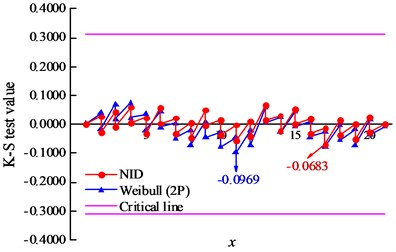a) Sample 1#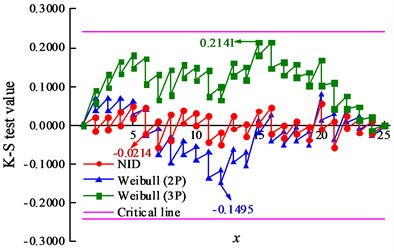b) Sample 2#

### 4.4. Comparison of goodness of fit

The K-S test is one of the most widely used goodness-of-fit tests . In this paper, the K-S test was used to discriminate the relative superiority of the NID distribution and Weibull distribution, and the differences between the empirical cumulative frequencies versus theoretical CDF values at every sample point are shown in Fig. 1. The maximum discrepancy of the K-S test results ${D}_{n}s$, critical values and cumulative probability values of the NID distribution and Weibull distribution are given in Table 5. The critical values of samples 1# and 2# are 0.3100 and 0.2420 under 95 % confidence level, respectively. The ${D}_{n}s$ results of the NID distribution are 0.0683 and 0.0576, and those of the Weibull distribution are 0.0969 and 0.1495, respectively. Clearly, both of the Weibull-type distributions pass the K-S testing, while the ${D}_{n}s$ of the NID distribution are much less than those of the Weibull distribution. In particular, the ${D}_{n}$ of the Weibull distribution is 2.6 times that of the NID distribution for sample 2#. In addition, both the cumulative probability values of the NID distribution are 1.0000. However, the cumulative probability values of the Weibull distribution are 0.9917 and 0.9966, respectively. It can be concluded that the fitting accuracy of the NID distribution is higher than that of the Weibull distribution.

### 4.5. Comparison of the fitting probability distribution curves

The empirical cumulative frequency curves and theoretical CDF curves for the two actual sample datasets are given Fig. 2. Within the truncated interval, the goodness of fit of the NID distribution is much more accurate than that of the Weibull distribution.

Fig. 2Comparative CDF curves of the actual sample datasets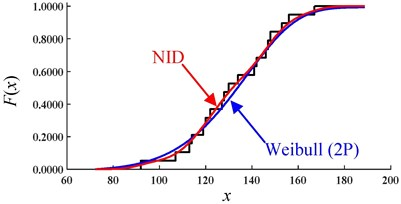a) Sample 1#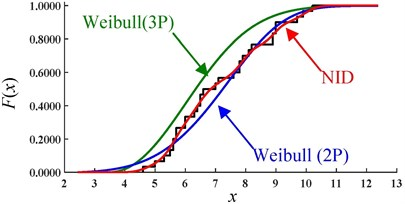b) Sample 2#

Fig. 3Comparative PDF curves of the actual sample datasets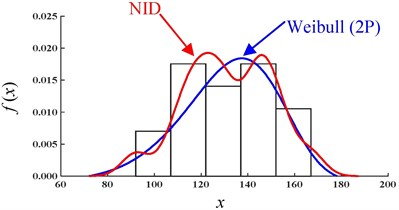a) Sample 1#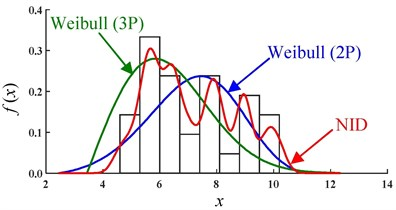b) Sample 2#

The PDF curves and histograms for the two actual samples are also given in Fig. 3. Due to the uncertainty in and complexity of the geotechnical parameters, the distributions of the actual samples often present a certain fluctuation. As one of the single-peak distributions, the Weibull distribution cannot be used to describe the characteristics of the fluctuation in the actual distribution. However, the NID distribution is very flexible and can be used to describe this fluctuation (Fig. 3).

To summarize, whether for CDF curves or PDF curves, the NID distribution will approximate the actual distribution more accurately than the Weibull distribution will. The superiority of the NID distribution can be further confirmed by describing the actual distributions of the geotechnical parameters.

## 5. Effect of sample size on fitting accuracy

Considering that the sample sizes obtained in actual geotechnical engineering are generally small, to study the effect of the sample size on the fitting accuracy with the NID distribution and Weibull distribution, eight simulated samples of different sizes were produced by using the MC method in this paper. Two known Weibull distributions estimated by samples 1# and 2#, WBL1# (7.2500, 140.3000) and WBL2# (5.0339, 7.7777), were used as the generating functions in the MC method, and the simulated sample sizes are 15, 20, 30, 50, 100, 200, 500, and 1000 (partial sample datasets are shown in Table 6).

The K-S test was first used to test the validity of the NID distribution and Weibull distribution. The K-S test results and critical values under different sizes are given in Table 7 and Table 8. The effect of the sample size on the K-S test results is shown in Fig. 4. With an increase in the sample size, the K-S test results of the two fitting methods gradually decrease and tend to converge to the horizontal axis. However, compared with the K-S test results of the Weibull distribution, those of the NID distribution are much lower. The convergence speed and stability and the K-S test results of the NID distribution are all superior to those of the Weibull distribution.

In addition, the chi-square test was used to investigate the fitting ability for all the samples with a sample size larger than 50. The chi-square test results for a 95 % confidence level are shown in Table 9.

Table 6Partial simulated samples with the MC method

 Size Simulated data 15 1# 122.1827, 111.8577, 111.4772, 144.3043, 143.4258, 132.1535, 142.5631, 111.0938, 113.1547, 130.3050, 146.0154, 122.9854, 147.7328, 135.6769, 139.4246 2# 5.6766, 4.9123, 8.9816, 4.8271, 6.6610, 9.1989, 8.1665, 7.0353, 4.1709, 4.0127, 8.7865, 3.8716, 4.1776, 7.2920, 5.7718 20 1# 131.3119, 72.4864, 117.7505, 81.6449, 147.6651, 131.8557, 163.0097, 117.6235, 127.7709, 108.4023, 76.0449, 97.8086, 138.1223, 186.8482, 131.1880, 149.3262, 148.6061, 142.5358, 157.7919, 118.3333 2# 9.1276, 4.0796, 10.8625, 5.9287, 5.6591, 5.2686, 9.3094, 7.6447, 8.2525, 5.7731, 7.5141, 4.8584, 8.6470, 8.2342, 8.8605, 8.9211, 5.2635, 6.8948, 7.0227, 8.8642 30 1# 118.2600, 131.0561, 141.8753, 111.0446, 130.5486, 91.0131, 103.9049, 140.8998, 130.8867, 141.4201, 126.5545, 114.3751, 118.4575, 155.1633, 112.0200, 167.9393, 137.8663, 119.5188, 115.6696, 140.3312, 118.5326, 103.9849, 147.2000, 154.8328, 148.2517, 141.2433, 144.6531, 98.2004, 163.0277, 128.3052 2# 5.3974, 6.7077, 7.8491, 7.1765, 7.6363, 9.3872, 8.3475, 9.0068, 8.6356, 8.3473, 7.5724, 9.6763, 4.9454, 4.3994, 7.2694, 7.2760, 7.9056, 4.9734, 7.7720, 9.0935, 5.8966, 7.6864, 8.3389, 7.6276, 9.2076, 8.9481, 4.4431, 4.1979, 6.9143, 9.5544 $⋮$ $⋮$ $⋮$

Table 7The K-S test results and CDF values of sample 1#

 Size Truncated interval ${D}_{n,0.05}$ K-S test results CDF values Left Right Weibull NID Weibull NID 15 85.4387 171.6572 0.3380 0.2607 0.1113 0.9596 1.0000 20 31.5233 216.2854 0.2940 0.1476 0.0799 1.0000 1.0000 30 71.3670 187.9536 0.2420 0.1388 0.0334 0.9923 1.0000 50 38.9533 196.7983 0.1923 0.1127 0.0200 0.9999 1.0000 100 46.1519 195.3722 0.1360 0.0894 0.0100 0.9997 1.0000 200 64.3473 193.7082 0.0962 0.0417 0.0050 0.9965 1.0000 500 59.6480 196.2190 0.0608 0.0487 0.0020 0.9980 1.0000 1000 56.5405 196.9676 0.0430 0.0301 0.0010 0.9986 1.0000

Table 8The K-S test results and CDF values of sample 2#

 Size Truncated interval ${D}_{n,0.05}$ K-S test results CDF values Left Right Weibull NID Weibull NID 15 0.4664 12.5077 0.3380 0.3336 0.0730 1.0000 1.0000 20 1.7339 12.8139 0.2940 0.1857 0.0500 0.9995 1.0000 30 1.5277 12.2911 0.2420 0.1865 0.0344 0.9997 1.0000 50 3.3735 11.1177 0.1923 0.1238 0.0200 0.9828 1.0000 100 1.9711 11.8769 0.1360 0.0552 0.0137 0.9988 1.0000 200 2.2851 11.8036 0.0962 0.0310 0.0050 0.9976 1.0000 500 1.9713 11.8592 0.0608 0.0475 0.0020 0.9988 1.0000 1000 2.2985 11.9048 0.0430 0.0209 0.0010 0.9976 1.0000

Fig. 4K-S test results of the simulated data with the sample size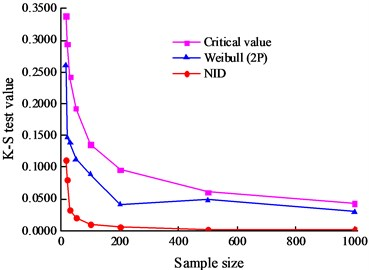a) Sample 1#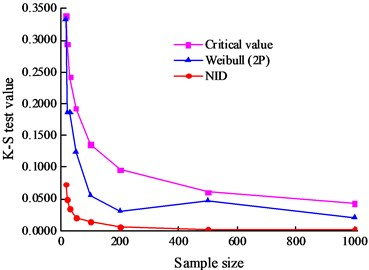b) Sample 2#

Table 9The results of the chi-square tests of samples 1# and 2#

 The sizes of MC data The number of intervals The chi-square test results Critical value for Weibull Weibull Critical value for NID NID 1# 50 7 9.4877 7.5426 12.5916 0.5208 100 10 14.0671 8.8863 16.9190 1.2689 200 14 19.6751 11.1422 22.3621 0.1825 500 22 30.1435 15.0571 32.6705 0.5096 1000 31 41.3372 30.2494 43.7729 0.6558 2# 50 7 9.4877 4.7083 12.5916 1.0037 100 10 14.0671 4.0272 16.9190 0.6340 200 14 19.6751 5.1070 22.3621 0.4919 500 22 30.1435 9.6412 32.6705 0.3132 1000 31 41.3372 28.9021 43.7729 0.5684

The change in the chi-square test results with an increase in the sample size are shown in Fig. 5 for the simulated samples. It can be seen that both the NID distribution and Weibull distribution have passed the chi-square test. However, the test results of the NID distribution are considerably lower than those of the Weibull distribution; the test results of the Weibull distribution are one to two orders of magnitude greater than those of the NID distribution. Thus, the goodness of fit of the NID distribution is superior to that of the Weibull distribution. Moreover, the test results of the NID distribution are much more stable than those of the Weibull distribution.

Fig. 5The chi-square test results of the simulated data with the sample size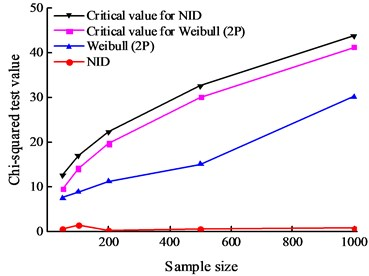a) Sample 1#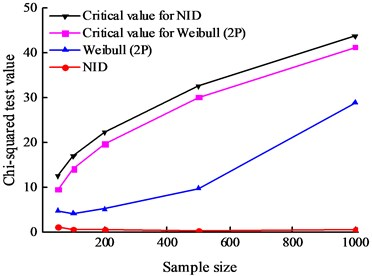b) Sample 2#

The CDF curves of the NID distribution and Weibull distribution for the simulated data of sample 1# are shown in Fig. 6. It is easy to see that, with an increase in the sample size, the CDF curves of the NID distribution are always closer to the empirical cumulative distribution function (EDF) curves than those of the Weibull distribution. Clearly, when the sample size is equal to 1000, the curves of the NID, Weibull and empirical distributions are nearly coincident.

Fig. 6Comparative CDF curves of the simulated data of sample 1# (sample size n)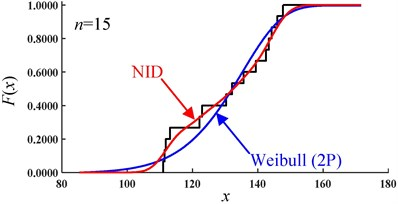a)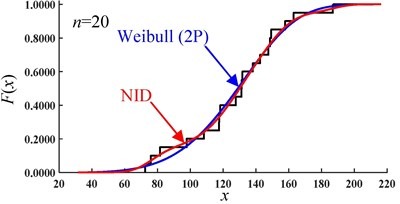b)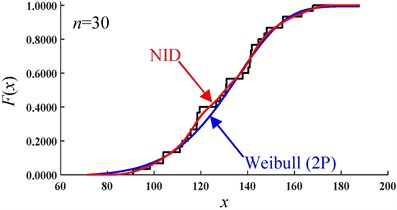c)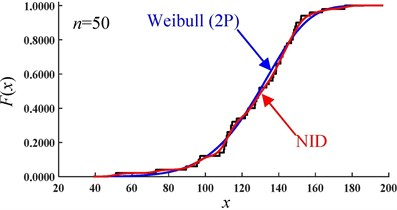d)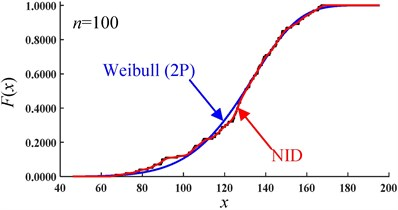e)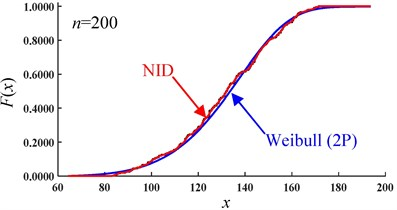f)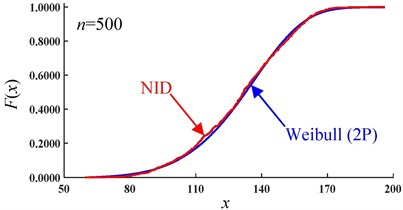g)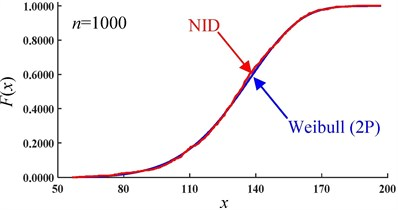h)

The CDF values for simulated samples 1# and 2# with different sizes are shown in Tables 7 and 8, and the trends of the CDF values with sample size are shown in Fig. 7. Clearly, with an increase in the sample size, the cumulative probability values of the NID distribution are always equal to 1.0000 and are completely unaffected by the sample size. However, the cumulative probability values of the Weibull distribution are generally less than 1.0000, and there is a considerable amount of volatility when the sample size increases. It is evident from the above analysis that the NID distribution has a higher fitting precision and wider applicability.

Fig. 7Cumulative probability values of the simulated data with the sample size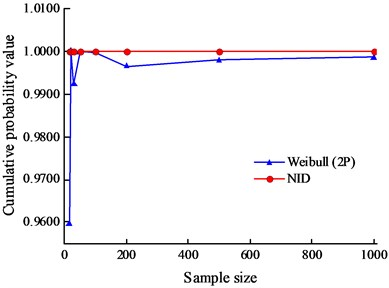a) Sample 1#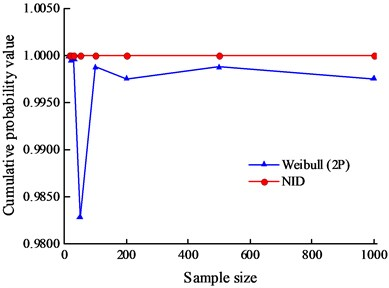b) Sample 2#

## 6. Discussion

In the truncated interval, the cumulative probability values of classical distributions are usually less than 1.0000. To solve this problem, the normalization of the truncated classical distribution was introduced. The basic principle of normalized distribution is introduced as follows:

5
6
$k=\frac{1}{F\left(R\right)-F\left(L\right)},$

where $\stackrel{~}{f}\left(x\right)$ is the normalized PDF, $F\left(x\right)$ is the cumulative PDF, $f\left(x\right)$ is the classical PDF, $x$ is the value of the sample, and $R$ and $L$ are the maximum and minimum values of the sample, respectively.

Table 10The results of K-S test values of the normalized Weibull distributions

 Sample ${D}_{n,0.05}$ $k$ Normalized Weibull NID Weibull Actual 1# 0.3100 1.0047 0.1046 0.0683 0.0969 2# 0.2420 1.0030 0.1475 0.0576 0.1495 MC 1# 15 0.3380 1.0038 0.1670 0.1113 0.2607 20 0.2940 1.0006 0.1031 0.0799 0.1476 30 0.2420 1.0065 0.1225 0.0334 0.1388 50 0.1923 1.0002 0.0736 0.0200 0.1127 100 0.1360 1.0005 0.0769 0.0100 0.0894 200 0.0962 1.0031 0.0428 0.0050 0.0417 500 0.0608 1.0027 0.0334 0.0020 0.0487 1000 0.0430 1.0018 0.0287 0.0010 0.0301 MC 2# 15 0.3380 1.0002 0.1579 0.0730 0.3336 20 0.2940 1.0008 0.1409 0.0500 0.1857 30 0.2420 1.0001 0.1091 0.0344 0.1865 50 0.1923 1.0051 0.0722 0.0200 0.1238 100 0.1360 1.0008 0.0494 0.0137 0.0552 200 0.0962 1.0014 0.0432 0.0050 0.0310 500 0.0608 1.0011 0.0292 0.0020 0.0475 1000 0.0430 1.0020 0.0207 0.0010 0.0209

The K-S test values of the normalized Weibull distribution for a 95 % confidence level are shown in Table 10. The sequence of the K-S test value of actual sample 1# is 0.0683 (NID) < 0.0969 (Weibull) < 0.1046 (normalized Weibull) < 0.3100 (Critical value). The sequence of the K-S test value of actual sample 2# is 0.0576 (NID) < 0.1475 (normalized Weibull) < 0.1495 (Weibull) < 0.2420 (Critical value). It can be found that all of the K-S test values pass the testing. However, all of the K-S test values of the normalized Weibull distribution are much more than those of the NID distribution, which indicates that the fitting ability of NID distribution is better than that of normalized Weibull distribution.

## 7. Conclusions

To accurately approximate the PDFs for geotechnical parameters, the NID method was introduced; several conclusions of this study are given below.

1) The PDFs of two sets of geotechnical samples were fitted with the NID distribution and Weibull distribution. The results show that, for the K-S test results, the chi-square test results and the cumulative probability values, the NID distribution is more accurate than the Weibull distribution. In addition, compared with the PDF curves of the Weibull distribution, those of the NID distribution can overcome the single-peak feature of the classical distributions and agree more closely with those of the actual distribution.

2) The effect of the sample size on the fitting accuracy for the NID distribution and Weibull distribution was investigated with the MC method, and eight simulated samples were produced. It can be found that with an increase in the sample size, the K-S test results of the NID distribution are all lower than those of the Weibull distribution. In addition, its convergence speed and stability are superior to those of the Weibull distribution. The cumulative probability values of the NID distribution are always equal to 1.0000 in the truncated interval and are unaffected by the sample size. However, the cumulative probability values of the Weibull distribution are generally less than 1.0000 and are unstable.

3) The comparison of the fitting accuracy between the NID distribution and the normalized Weibull distribution was also discussed, and the results show that, even if the cumulative probability values are equal to 1 for those two distributions, the fitting accuracy of the NID distribution is still higher than that of normalized Weibull distribution.

#### Acknowledgements

This research was financially supported by the Natural Science Foundation of China (Grant No. 41102170) and the Fundamental Research Funds for the Central Universities of Central South University (Grant No. 2017zzts536).